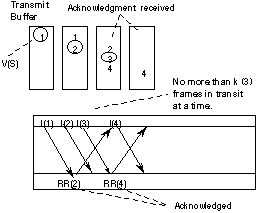# HDLC Sequence Numbers &Windows

Two sequence numbers are employed by HDLC when providing a reliable data link service. One sequence number, the send sequence number (N(S)) records the number of each PDU sent. Another sequence number returns an acknowledgment confirming correct delivery of data to the receiver. This is the receive sequence number (N(R)).

### The send sequence number

The send sequence number is the number (in the specified modulus) of the current I-frame being sent by the local node. Each transmitted I-frame is numbered in succession with a sequence number. This is implemented by copying the send state variable, V(S), into the send sequence number, N(S), in the frame. After a frame is transmitted the send state variable is incremented. The N(S) in retransmitted frames are not changed (i.e. the original N(S) value is sent in the retransmitted frame).

The receive sequence number is the number (in the same modulus) of the next I-frame expected by the node at the remote end of the link. For a link with no errors, the N(R) of the next frame sent by the remote node should be one more than the N(S) of the last frame received by the node. The N(R) value is equal to the remote node's receive sequence variable, V(R). Since the reception of a valid in-sequence I-frame causes the V(R) value to be assigned one plus the last N(S) value, the received N(S) value is one greater than the sent N(R) value. This process is known as "acknowledgement" and indicates to the local node that the remote node has correctly received all I-frames sent with a N(S) sequence number less than the received N(R) value (i.e. it is said to be "culmulative").

### Windows

Many protocols need to number the PDUs which are sent by the protocol in order to provide a reliable service. Usually this numbering is performed by including sequence numbers in the Protocol Control Information (PCI) (i.e. the PDU header). The sequence numbers are normally stored using modulo-n arithmetic allowing the same set of numbers to be used time after time. A technique known as a "window" is used to ensure that the same sequence number is never used by two frames at any one time.

### Example of the use of a Window in HDLC

An HDLC link (operating in the ABM mode) connecting two equipments A and B is operated with a window (k) of 3 frames. Part of the transmission is shown in the frame transition diagram below. This diagram will be used to explain the terms modulus and window size.Exchange of frames from A to B illustrating the operation of the HDLC window

The modulus is used to specify the numbering to be used for all internal state variables and sequence numbers. HDLC specifies two moduli (8 and 128) allowing sequence numbers in the range 0-7 and 0-127 respectively. Recent additions to the standards also allow larger moduli for special applications. The wrapping of the send sequence number in the diagram shown, indicates that a modulus of 8 is being used.

The window specifies the maximum number of frames which may be transmitted without receiving an acknowledgment. The current window is defined as the number of frames which may be sent at the current time, this is always less than or equal to the link window size. the diagram above shows a window size of 3 frames. This results in a pause in transmission after sending the third frame, until the first frame has been acknowledged by the remote node (B). The window size also defines the amount of buffering required at the sending node (A) to hold one copy of each frame which is unacknowledged. (These may subsequently be retransmitted if no acknowledgment is received or a retransmission is explicitly requested using a REJ frame).

The diagram below shows the operation of the window as viewed by the transmitter (A). The upper horizontal line represents system A and the lower, system B. Time increases from left to right. The numbers in the format used in the frame transition diagram represent the send sequence number, N(S), and the receive sequence number, N(R), carried in the HDLC frame header.Operation of the transmitter for a HDLC link with a window size of 3

Gorry Fairhurst - Date: 01/10/2001 EG3557